Next: Secular perihelion precession rate Up: Perihelion precession of Mercury Previous: Perihelion precession rate

# Evaluation of disturbing function

It is convenient to evaluate the disturbing function,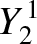(B.23)

in a frame of reference that is instantaneously aligned with the ecliptic plane, as described in Section 4.12. Let (,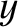,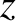) and (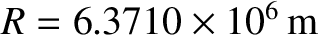,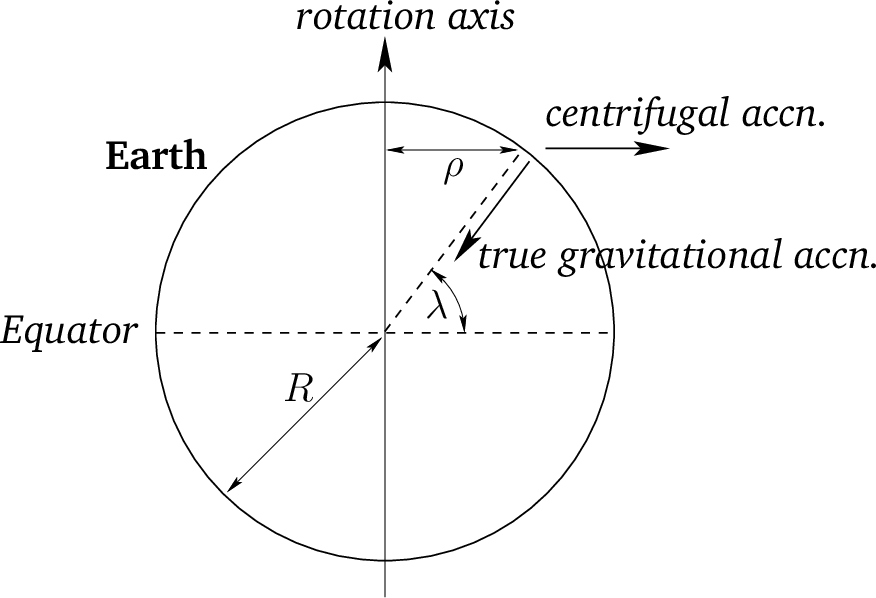,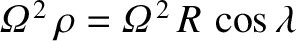) be the Cartesian components of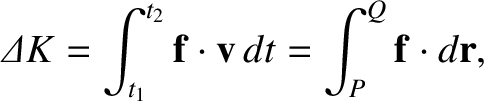and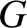, respectively, in this standard reference frame. It follows from Equations (4.38), and (4.72)-(4.74), that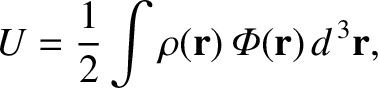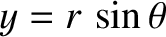(B.24)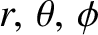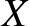(B.25) and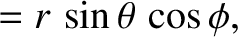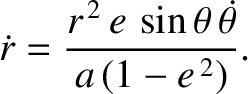(B.26)

as well as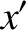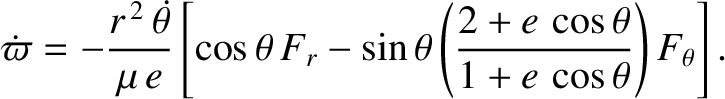(B.27)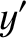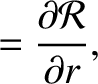(B.28) and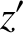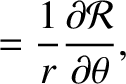(B.29)

where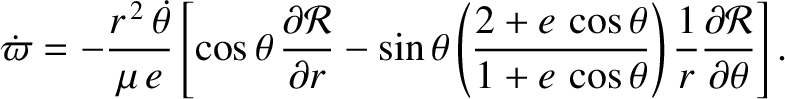(B.30) and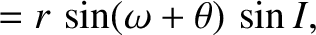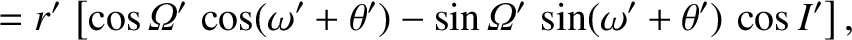(B.31)

Here,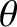,,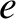,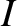,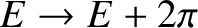, and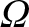are the orbital true anomaly, major radius, eccentricity, inclination, argument of the perihelion, and longitude of the ascending node, respectively, of Mercury. Moreover,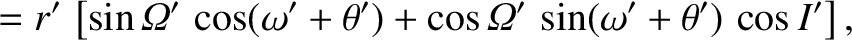,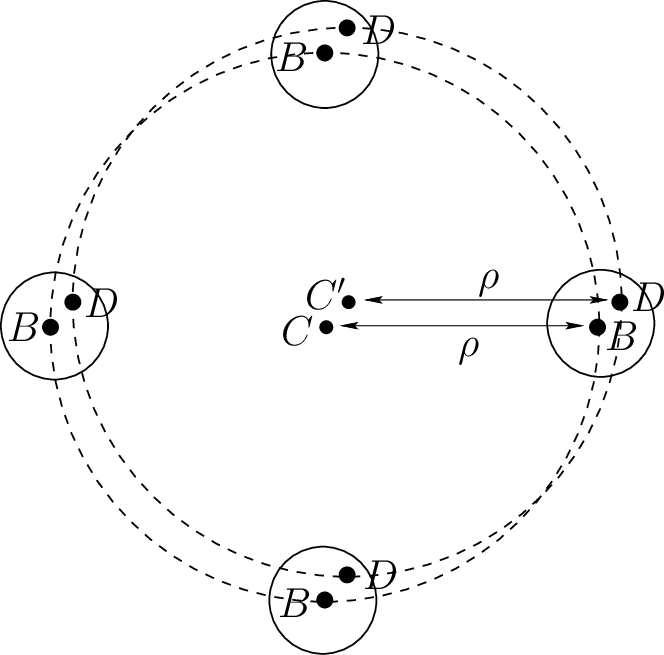,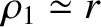,,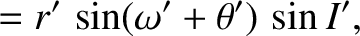, and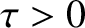are the corresponding quantities for the perturbing planet.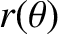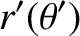(B.32) and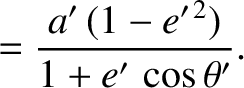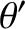(B.33)

Making use of Equations (B.25)-(B.30), we deduce that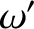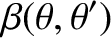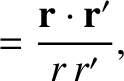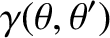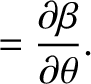(B.34)

and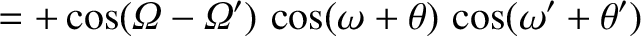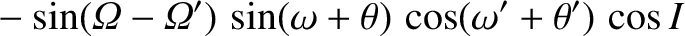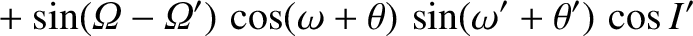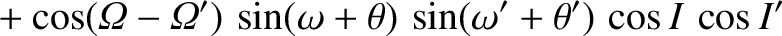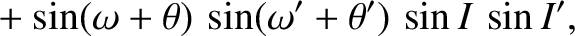(B.35)

Hence,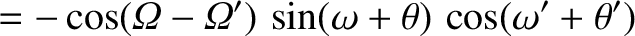(B.36)

which implies that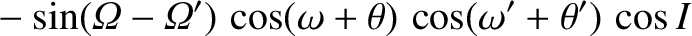(B.37)

and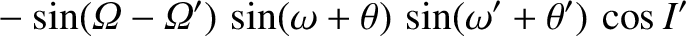(B.38)Next: Secular perihelion precession rate Up: Perihelion precession of Mercury Previous: Perihelion precession rate
Richard Fitzpatrick 2016-03-31